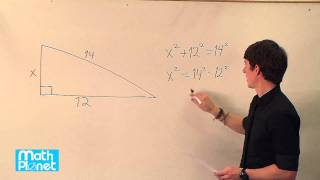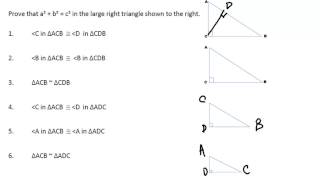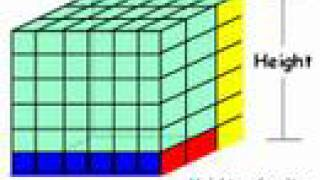# The converse of the Pythagorean theorem and special triangles

##By MathPlanetVideos

Find the value of x in the right triangle.# Pythagorean theorem 3 | Right triangles and trigonometry | Geometry | Khan Academy

##By Khan Academy

This three-minute video is from Sal Khan about using the Pythagorean Theorem to solve a word problem: A carpet measures 7 feet long and has a diagonal measurement of square root of 74 feet. Find the width of the carpet Mr. Khan uses computer software for demonstration. The screen is a little 'busy' so the viewer may want to open the screen to 'full screen' to see all more clearly.# Proof of Pythagorean Theorem using similar triangles

##By Matt Stryker

Proof of Pythagorean Theorem using similar triangles# Volume of Rectangular Prisms: Length Width and Height

##By Have Fun Teaching

The Volume Song and movie teaches students how to find the volume of a solid figure. Learn how to solve and find the volume of a 3D rectangular prism shape using length times width times height.# Another Pythagorean theorem proof | Right triangles and trigonometry | Geometry | Khan Academy

##By Khan Academy

Khan Academy presents Another Pythagorean Theorem Proof, an educational video resource on math.# Pythagorean theorem 1 | Right triangles and trigonometry | Geometry | Khan Academy

##By Khan Academy

This is a Khan Academy video about solving a word problem involving the need to find the length of a rope that attaches a mast of a boat with the deck of the boat.# Pythagorean theorem 2 | Right triangles and trigonometry | Geometry | Khan Academy

##By Khan Academy

In this video, Sal Khan continues discussing the Pythagorean Theorem to solve for the third side of the triangle Mr. Khan uses computer software for demonstration. The screen is a little 'busy' so the viewer may want to open the screen to 'full screen' to see all more clearly.# The Pythagorean theorem intro | Right triangles and trigonometry | Geometry | Khan Academy

##By Khan Academy

Watch this video to review right triangles and the Pythagorean Theorem view an example of using the Pythagorean Theorem to find the length of a hypotenuse (the longest side of a right triangle) view an example of using the Pythagorean Theorem to find the length of a leg of a right triangle